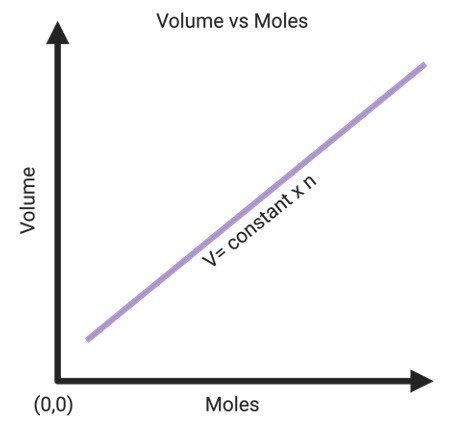Research Tweet

In 1811, Avogadro’s law was described by a famous Italian chemist and physicist named Amedeo Avogadro. Avogadro’s law is a relationship between volume of gas and the number of moles.

This law states that at a constant temperature and pressure the total number of atoms or molecules present in a gas is directly proportional to the volume occupied by that gas.

This equation is given by;

V ∝ n

V=kn or k = V/n

V = volume of gas

n = Number of moles present in given gas

k = proportionality constant

The graphical representation of Avogadro’s law is given below;The straight line indicates that the two quantities that are volume and number of moles present in gas are directly proportional to each other.

Thus, the straight line that passes through the origin signifies that 0 moles for gas will occupy zero space.

Avogadro’s law can be derived from the ideal gas equation, which can be written as follows:

PV = nRT

• ‘P’ is defined as the pressure applied by the gas on the walls of container

• ‘V’ is defined as the volume occupied by the gas

• ‘n’ is defined as number of moles of gas present in given molecule

• ‘R’ is defined as the universal gas constant

• ‘T’ is defined as the absolute temperature of the gas

By reorganizing the ideal gas equation, the following equation can be obtained.

V/n = (RT)/P

Here, the value of (RT)/P is constant because pressure and temperature are constant according to Avogadro’s hypothesis and R is the universal constant.

Therefore,

V/n = k

Thus, the proportionality between the volume occupied by a gas and the number of molecules present in given gas is confirmed.

Given below are few examples of Avogadro’s law;

###### I. Breathing

One of the finest example of Avogadro’s law is breathing. When we inhale, our lungs enlarge because they are filled with air. Similarly, when we exhale, the lungs let the air out and thus shrink in size. This change in volume is observed, which is proportional to the amount or the number of molecules of air confined by the lungs.

###### II. Inflating Tyres

The shape of a flat tyres gets distorted in the absence of air inside it. As soon as the flat tyres is filled with the required amount of air, it gets back to its original shape. Hence, the inflation of flat tyres is a clear example of Avogadro’s law in our everyday life.

###### III. Bicycle Pump Action

The pump abstracts the air from the environment and pushes it inside a flattened object. This increase in the amount of gas molecules in the object congruently changes its shape and helps it to enlarge. This example is clearly explained by Avogadro’s law.

###### IV. Pool Tube

A flattened pool tube becomes transportable as the number of air particles inside the tube is decreased which in turn reduces its volume and makes it compact.

During inflation, when the tube is filled with air, increasing the number of air molecules in it which in turn increases the volume and size of the pool tube. Henceforth, Avogadro’s law can be applied to inflate or deflate the given pool tube as per our necessity.

Avogadro’s number is defined as the number of molecules of gas present in one mole that is huge (6.02×1023).

The unit for Avogadro number is mole-1.

Avogadro’s number is generally symbolized by N.

Avogadro’s number is a unit used by chemists for easy calculations all over the world.

After realizing that the volume of a gas is directly proportional the number of particles present in the gaseous molecules, this formula established a vital relationship for simple molecules at that time when the distinction between atoms and molecules was not visibly understood.

Specifically, the existence of diatomic molecules such as that H2, O2 and Cl2 was not identified until the results of researches involving the volume of gas molecules was discovered.

This law is also known as Avogadro’s principle or Avogadro’s hypothesis. This law is only relevant to ideal gases and gives an estimated result for the real gases.

The gases with light molecules for instance helium, hydrogen, etc., follow Avogadro’s law more precisely as compared to the gases with heavy molecules.

Share

###### Similar Post: